Function Repository Resource:

# CurveTorsion

Compute the torsion of a curve

Contributed by: Wolfram Staff (original content by Alfred Gray)
 ResourceFunction["CurveTorsion"][c,t] computes the torsion of a space curve c parametrized by t.

## Details and Options

Torsion gives a measure of how rapidly a curve deviates from its osculating plane.

## Examples

### Basic Examples (4)

Plot the twisted cubic curve:

 In:=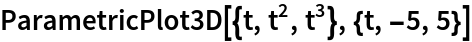Out=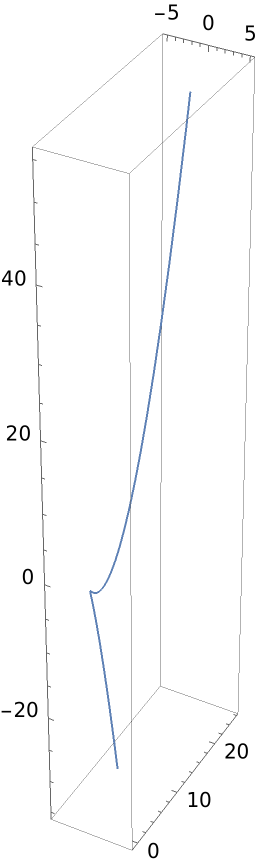Compute the torsion of the twisted cubic curve:

 In:=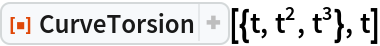Out=Compute the curvature using the resource function Curvature:

 In:=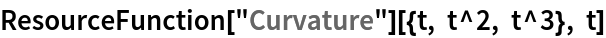Out=Plot them:

 In:=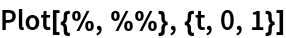Out=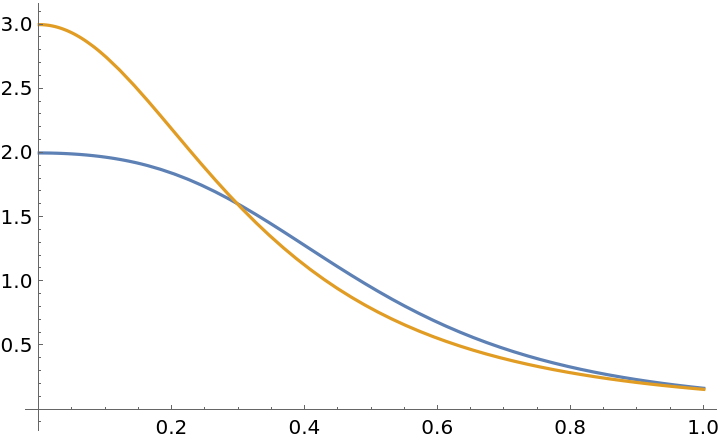### Scope (2)

For this curve, the torsion and curvature are the same:

 In:=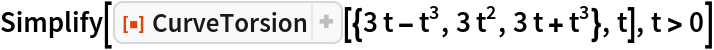Out=In:=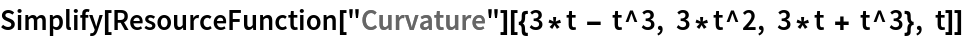Out=Plot of the above results:

 In:=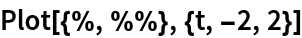Out=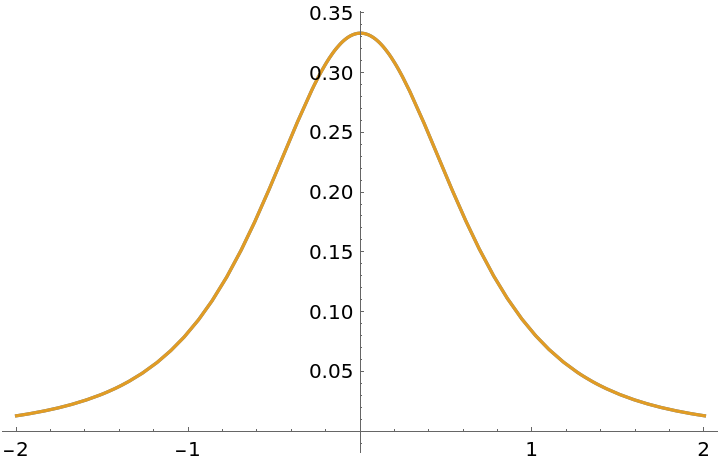A curve that is qualitatively similar to a torus knot:

 In:=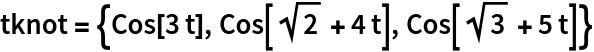Out=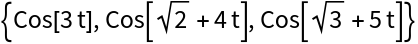Plot the curve:

 In:=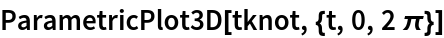Out=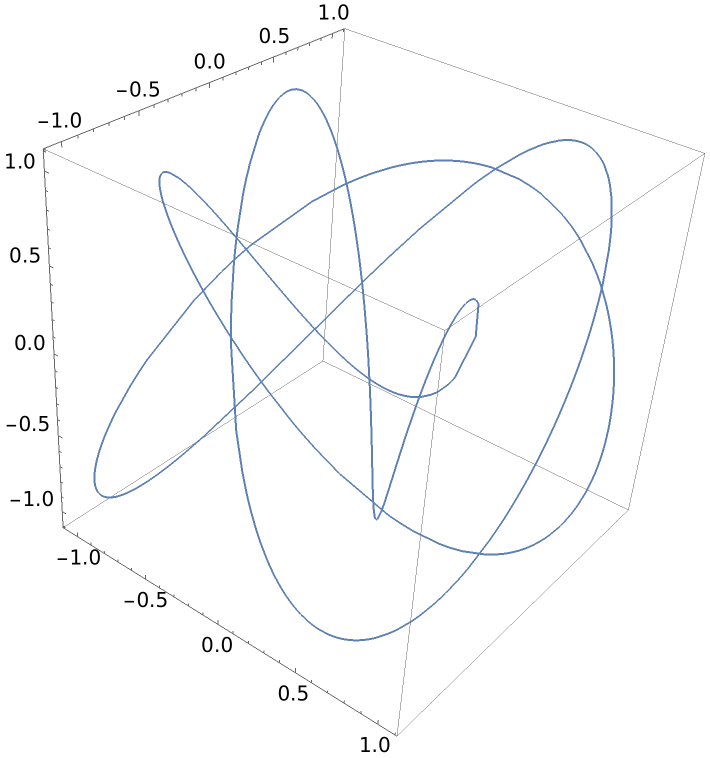Find the torsion:

 In:=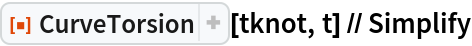Out=Plot this:

 In:=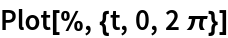Out=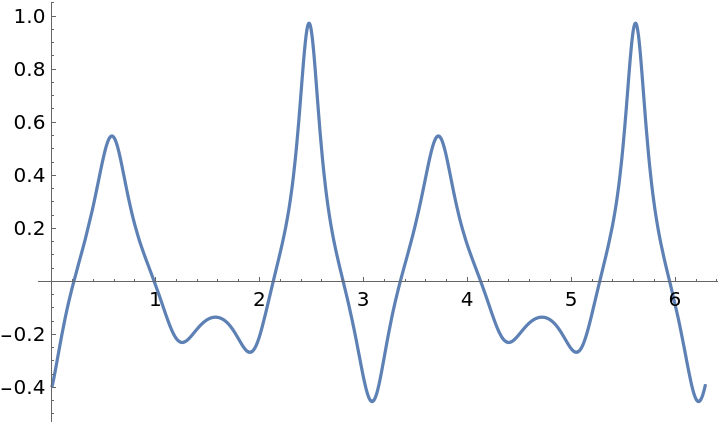Compute the curvature with the resource function Curvature:

 In:=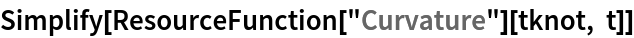Out=In:=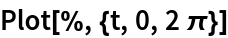Out=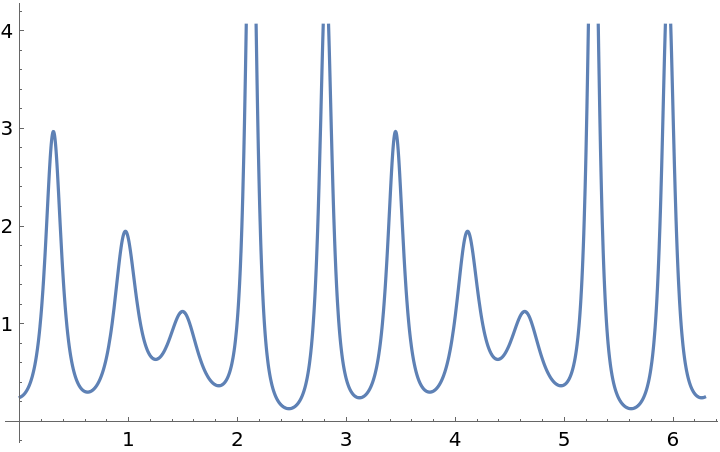### Properties and Relations (2)

Define a conical spiral:

 In:=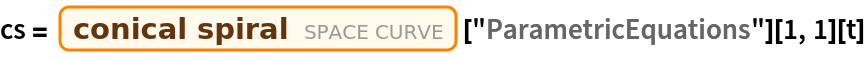Out=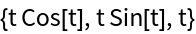Here is the torsion:

 In:=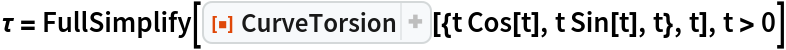Out=There are other quantities related to torsion. The inverse of the torsion is called the radius of torsion:

 In:=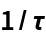Out=The curvature, which can be calculated with the resource function Curvature:

 In:=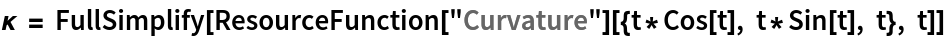Out=There is also the so-called total curvature:

 In:=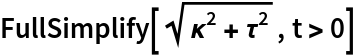Out=Definition of a unit speed helix:

 In:=The curvature, via the resource function Curvature:

 In:=Out=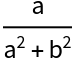The torsion:

 In:=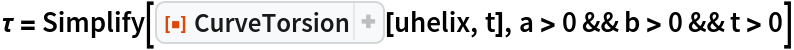Out=The relation to the Frenet-Serret system is that the curvature and the torsion are the first two quantities:

 In:=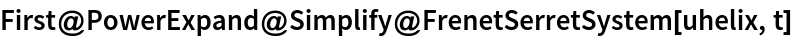Out=Enrique Zeleny

## Version History

• 1.0.0 – 30 April 2020